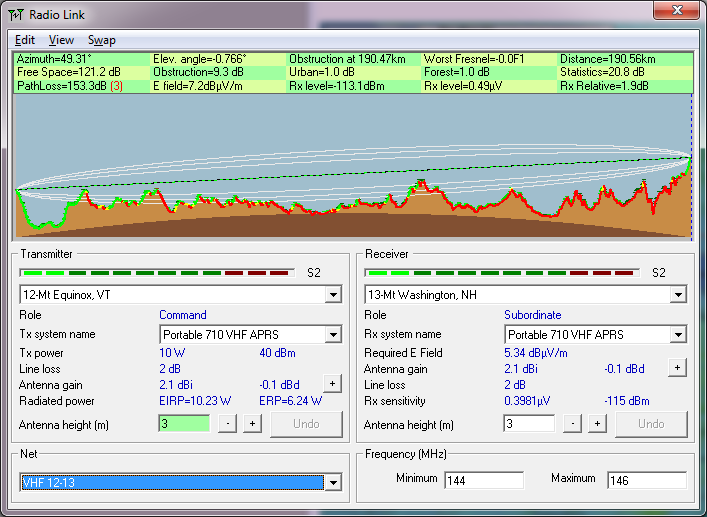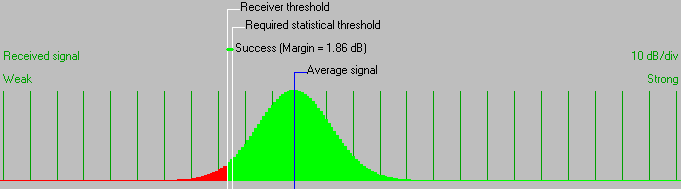• Distance between 12-Mt Equinox, VT and 13-Mt Washington, NH is 190.6 km (118.4 miles)
• True North Azimuth = 49.31°, Magnetic North Azimuth = 63.39°, Elevation angle = -0.7658°
• Terrain elevation variation is 1778.1 m
• Propagation mode is scatter, single obstruction, 0.0F1 at 190.5km
• Average frequency is 145.000 MHz
• Free Space = 121.2 dB, Obstruction = 9.3 dB, Urban = 1.0 dB, Forest = 1.0 dB, Statistics = 20.8 dB
• Total propagation loss is 153.3 dB (interference mode (optimistic))
• System gain from 12-Mt Equinox, VT to 13-Mt Washington, NH is 155.2 dB
• System gain from 13-Mt Washington, NH to 12-Mt Equinox, VT is 155.2 dB
• Worst reception is 1.9 dB over the required signal to meet 95.000% of situations
• Warning 3